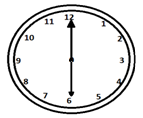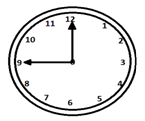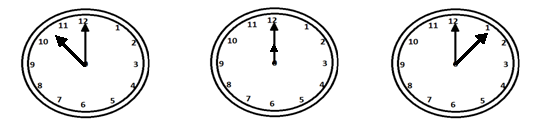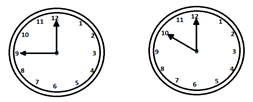•••••••••••••••••••••••••••••••••••••••••••••••••••••••••••••••••••••••••••••••••••• Home /Exam Details (QP Included) / Preliminary Exam / General Mental Ability / W.B.C.S. Reasoning – Clock – W.B.C.S. Exam Short Tricks For Reasoning Questions.
•Posted on April 3rd, 2019 in General Mental Ability
Tags:

## W.B.C.S. Reasoning – Clock – W.B.C.S. Exam Short Tricks For Reasoning Questions.

In every Competitive exam specially in WBCS Examination, in both preliminary and W.B.C.S. main exam, one of the most important section is Reasoning. You can score a great marks in competitive exams, if you get a good score in Reasoning test. You can achieve a good marks only if you have a depth knowledge of reasoning skills. Logically and type wise reasoning can be divided into few more sections. Calendar is one of them. Continue reading W.B.C.S. Exam Short Tricks For Reasoning Questions On Clock.

We provide so many shortcut reasoning tricks and online mock tests. You need to learn those shortcut tricks carefully. You need to learn every topic of reasoning. Then purchase online PDF on reasoning or purchase reasoning books from the market. You can also download reasoning eBooks from online if it’s free. Then find out the practice sets in those books and solve those reasoning MCQ questions using tricks which you have learn here. This will show you the difference between usage of reasoning tricks and non usage of reasoning tricks. Our reasoning notes on Mathematical Operations will be your key success to your exam.

Now, we will discuss few important reasoning question answer and also discuss chapter Clock in detail.

### Basics of Clock

The hands of a clock can have a maximum angle of 180o between them. When this happens, both the hands represent a straight line.The hands of a clock make right angles twice every hour when they are 15 minutes away from each other.The two hands of a clock coincide once in every hour.A clock is very essential for every human being to schedule their daily tasks. The clock theory is related to everyday life.

A clock is an instrument that displays time by dividing it into hours, minutes, and seconds.

### Dial

It has a circular dial numbered from 1 to 12 that indicates the hours. The circumference of a dial is further divided into 60 equal spaces, that are called the minutes’ spaces.

1 hour = 60 minutes

1 minute = 60 seconds and

1 hour = 3600 seconds

### Tricks

• Between n and (n+1) Oclock, the two hands of a clock will coincide at (60n11)(60n11) min past n.
• Between n and (n+1) Oclock, the two hands of a clock will mutually make a right angle at (5n±15)×1211(5n±15)×1211 min past n.
• Between n and (n+1) Oclock, the hands of a clock will be in a straight line (without being together) at
• (5n30)×1211(5n−30)×1211 min past n,(when n >6)
• (5n+30)×1211(5n+30)×1211 min past n,(when n <6)
• Between n and (n+1) Oclock, the hands of a clock are xx min apart at (5n±x)×1211(5n±x)×1211 min past n. ‘+’ sign indicates that the minute hand is ahead and ‘–‘ sign indicates that the hour hand is ahead.
• If the minute hand of a clock overtakes the hour hand at an interval of xx min of the correct time, then the clock loses or gains by (72011x)(60×24x)(72011−x)(60×24x) min.

1 − At what time between 7.15 and 8.15, the hands of a clock will coincide each other?

Options −

A – 3951139511

B – 3941139411

C – 3851138511

D – 3941139411

Explanation −

60×n11=6011×294=3951160×n11=6011×294=39511

Where n=7.15=71560=294n=7.15=71560=294

2 − In what particular time, between 9 and 8 O’clock, both the hands will be at a right angle to each other?

Options −

A – 6541165411 and 3271132711 min past 9

B – 6521165211 and 3221132211 min past 9

C – 6531165311 and 3231132311 min past 9

D – 6511165111 and 3211132111 min past 9Explanation −

(5n±15)×1211=(5×9±15)×1211(5n±15)×1211=(5×9±15)×1211

=65411=65411 and 3271132711 min past 9

3 − In between 12 and 1 O`clock, when will both the hands of a clock form a straight line?

Options −

A – 3601136011 min. past 12

B – 3551135511 min. past 12

C – 3401134011 min. past 12

D – 3451134511 min. past 12

Explanation −

(5n30)×1211(5n−30)×1211 min past n

(630)×1211(6−30)×1211 min. past 12

=34511=34511 min. past 12 (since n = 12)

4 − In how much time, hands of a clock will be in the 30 min. space apart when they are in between 12 and 1 p.m. on Monday?

Options −

A – 108011108011 and 3001130011 min past 12

B – 108012108012 and 3601136011 min past 12

C – 108011108011 and 3601136011 min past 12

D – 108012108012 and 3001230012 min past 12

Explanation −

(5n±x)×1211(5n±x)×1211

=(5×12±30)×1211=(5×12±30)×1211

=90×1211=90×1211 and 30×121130×1211 min past 12 = 108011108011 and 3601136011 min past 12

5 − The minute hand of a clock overtakes the hour hand at interval of 61 min. then the clock loses or gain by how much time?

Options −

A – 10446711044671 min.

B – 10516711051671 min.

C – 10436711043671 min.

D – 10546711054671 min.

Explanation −

(7201161)×(60×2461)(72011−61)×(60×2461)

=1051671=1051671 min.